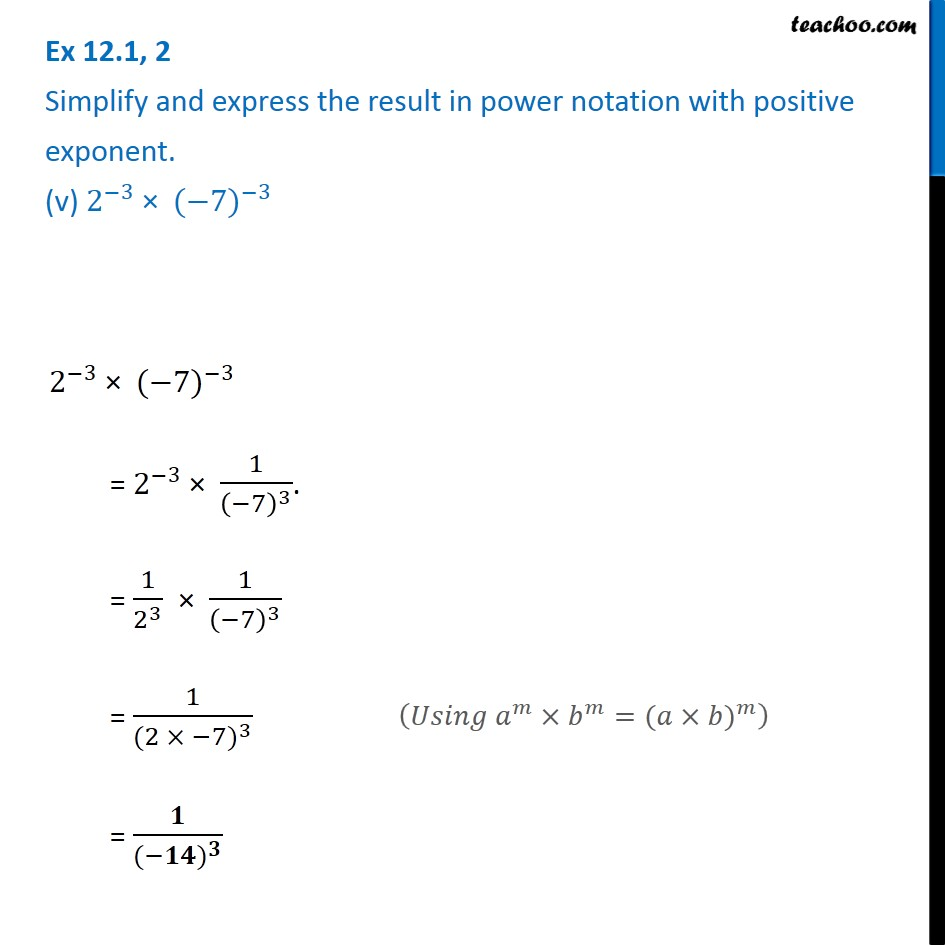Ex 12.1

Chapter 12 Class 8 Exponents and Powers
Serial order wiseGet live Maths 1-on-1 Classs - Class 6 to 12

### Transcript

Ex 12.1, 2 Simplify and express the result in power notation with positive exponent. (v) 2^(−3) × 〖(−7)〗^(−3) 2^(−3) × 〖(−7)〗^(−3) = 2^(−3) × 1/(−7)^3 . = 1/2^3 × 1/(−7)^3 = 1/((〖2 × −7)〗^3 ) = 𝟏/((〖−𝟏𝟒)〗^𝟑 ) (𝑈𝑠𝑖𝑛𝑔 𝑎^𝑚×𝑏^𝑚=〖(𝑎×𝑏)〗^𝑚 )